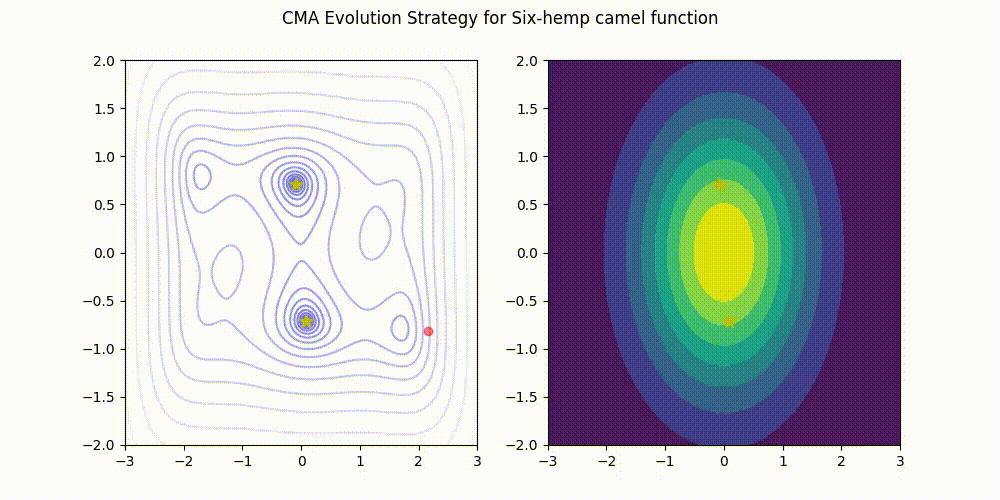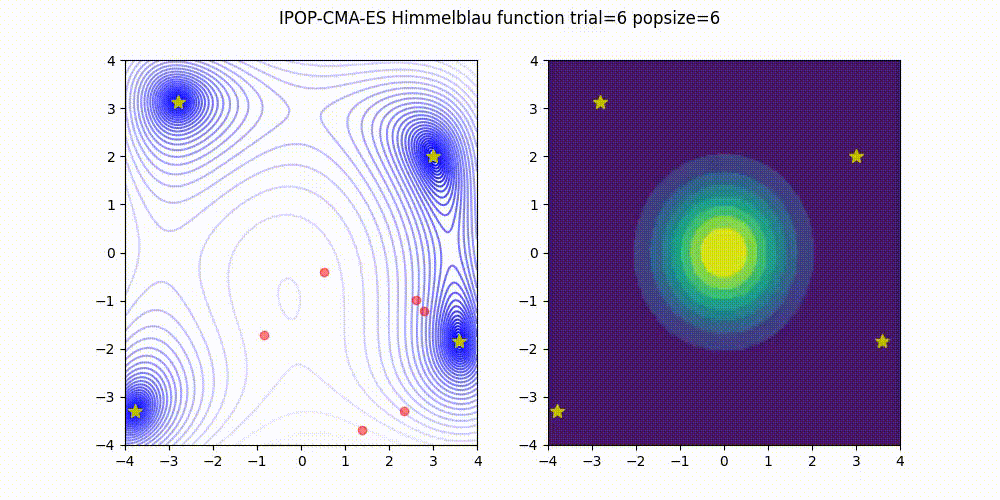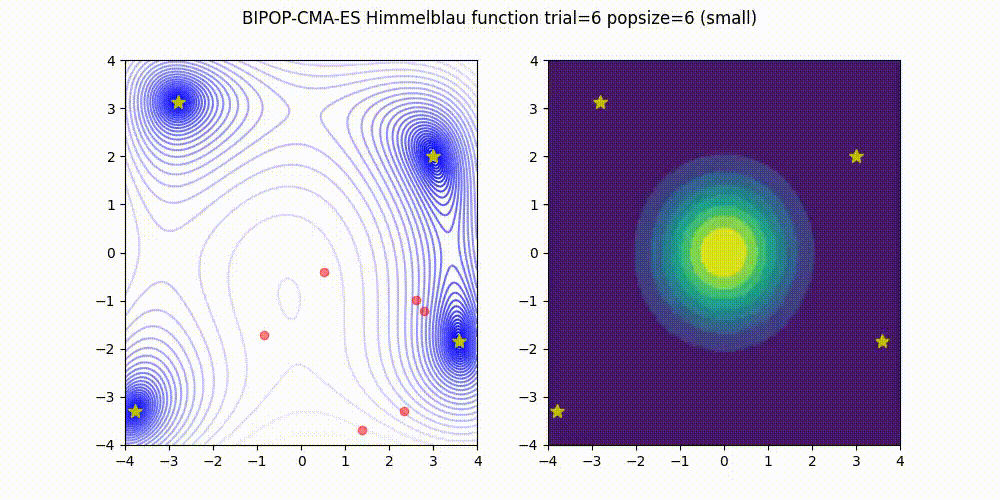# cmaes Release 0.8.2

Lightweight Covariance Matrix Adaptation Evolution Strategy (CMA-ES) implementation for Python 3.

Keywords
cma-es, evolution-strategy, hyperparameter-optimization, optuna
MIT
Install
``` pip install cmaes==0.8.2 ```

# CMA-ES

Lightweight Covariance Matrix Adaptation Evolution Strategy (CMA-ES)  implementation.## Installation

Supported Python versions are 3.7 or later.

``````\$ pip install cmaes
``````

Or you can install via conda-forge.

``````\$ conda install -c conda-forge cmaes
``````

## Usage

This library provides an "ask-and-tell" style interface.

```import numpy as np
from cmaes import CMA

return (x1 - 3) ** 2 + (10 * (x2 + 2)) ** 2

if __name__ == "__main__":
optimizer = CMA(mean=np.zeros(2), sigma=1.3)

for generation in range(50):
solutions = []
for _ in range(optimizer.population_size):
solutions.append((x, value))
print(f"#{generation} {value} (x1={x}, x2 = {x})")
optimizer.tell(solutions)```

And you can use this library via Optuna , an automatic hyperparameter optimization framework. Optuna's built-in CMA-ES sampler which uses this library under the hood is available from v1.3.0 and stabled at v2.0.0. See the documentation or v2.0 release blog for more details.

```import optuna

def objective(trial: optuna.Trial):
x1 = trial.suggest_uniform("x1", -4, 4)
x2 = trial.suggest_uniform("x2", -4, 4)
return (x1 - 3) ** 2 + (10 * (x2 + 2)) ** 2

if __name__ == "__main__":
sampler = optuna.samplers.CmaEsSampler()
study = optuna.create_study(sampler=sampler)
study.optimize(objective, n_trials=250)```

## CMA-ES variants

#### CMA-ES with Margin 

CMA-ES with Margin introduces a lower bound on the marginal probability associated with each discrete dimension so that samples can avoid being fixed to a single point. It can be applied to mixed spaces of continuous (float) and discrete (including integer and binary).

The above figures are taken from EvoConJP/CMA-ES_with_Margin.

Source code
```import numpy as np
from cmaes import CMAwM

def ellipsoid_onemax(x, n_zdim):
n = len(x)
n_rdim = n - n_zdim
r = 10
if len(x) < 2:
raise ValueError("dimension must be greater one")
ellipsoid = sum([(1000 ** (i / (n_rdim - 1)) * x[i]) ** 2 for i in range(n_rdim)])
onemax = n_zdim - (0.0 < x[(n - n_zdim) :]).sum()
return ellipsoid + r * onemax

def main():
binary_dim, continuous_dim = 10, 10
dim = binary_dim + continuous_dim
bounds = np.concatenate(
[
np.tile([-np.inf, np.inf], (continuous_dim, 1)),
np.tile([0, 1], (binary_dim, 1)),
]
)
steps = np.concatenate([np.zeros(continuous_dim), np.ones(binary_dim)])
optimizer = CMAwM(mean=np.zeros(dim), sigma=2.0, bounds=bounds, steps=steps)
print(" evals    f(x)")
print("======  ==========")

evals = 0
while True:
solutions = []
for _ in range(optimizer.population_size):
value = ellipsoid_onemax(x_for_eval, binary_dim)
evals += 1
solutions.append((x_for_tell, value))
if evals % 300 == 0:
print(f"{evals:5d}  {value:10.5f}")
optimizer.tell(solutions)

if optimizer.should_stop():
break

if __name__ == "__main__":
main()```

Source code is also available here.

#### Warm Starting CMA-ES 

Warm Starting CMA-ES is a method to transfer prior knowledge on similar HPO tasks through the initialization of CMA-ES. Here is the result of an experiment that tuning LightGBM for Kaggle's Toxic Comment Classification Challenge data, a multilabel classification dataset. In this benchmark, we use 10% of a full dataset as the source task, and a full dataset as the target task. Please refer the paper and/or https://github.com/c-bata/benchmark-warm-starting-cmaes for more details of experiment settings.Source code
```import numpy as np
from cmaes import CMA, get_warm_start_mgd

def source_task(x1: float, x2: float) -> float:
b = 0.4
return (x1 - b) ** 2 + (x2 - b) ** 2

def target_task(x1: float, x2: float) -> float:
b = 0.6
return (x1 - b) ** 2 + (x2 - b) ** 2

if __name__ == "__main__":
# Generate solutions from a source task
source_solutions = []
for _ in range(1000):
x = np.random.random(2)
source_solutions.append((x, value))

# Estimate a promising distribution of the source task,
# then generate parameters of the multivariate gaussian distribution.
ws_mean, ws_sigma, ws_cov = get_warm_start_mgd(
source_solutions, gamma=0.1, alpha=0.1
)
optimizer = CMA(mean=ws_mean, sigma=ws_sigma, cov=ws_cov)

# Run WS-CMA-ES
print(" g    f(x1,x2)     x1      x2  ")
print("===  ==========  ======  ======")
while True:
solutions = []
for _ in range(optimizer.population_size):
solutions.append((x, value))
print(
f"{optimizer.generation:3d}  {value:10.5f}"
f"  {x:6.2f}  {x:6.2f}"
)
optimizer.tell(solutions)

if optimizer.should_stop():
break```

The full source code is available here.

#### Separable CMA-ES 

sep-CMA-ES is an algorithm which constrains the covariance matrix to be diagonal. Due to the reduction of the number of parameters, the learning rate for the covariance matrix can be increased. Consequently, this algorithm outperforms CMA-ES on separable functions.

Source code
```import numpy as np
from cmaes import SepCMA

def ellipsoid(x):
n = len(x)
if len(x) < 2:
raise ValueError("dimension must be greater one")
return sum([(1000 ** (i / (n - 1)) * x[i]) ** 2 for i in range(n)])

if __name__ == "__main__":
dim = 40
optimizer = SepCMA(mean=3 * np.ones(dim), sigma=2.0)
print(" evals    f(x)")
print("======  ==========")

evals = 0
while True:
solutions = []
for _ in range(optimizer.population_size):
value = ellipsoid(x)
evals += 1
solutions.append((x, value))
if evals % 3000 == 0:
print(f"{evals:5d}  {value:10.5f}")
optimizer.tell(solutions)

if optimizer.should_stop():
break```

Full source code is available here.

#### IPOP-CMA-ES 

IPOP-CMA-ES is a method to restart CMA-ES with increasing population size like below.Source code
```import math
import numpy as np
from cmaes import CMA

def ackley(x1, x2):
# https://www.sfu.ca/~ssurjano/ackley.html
return (
-20 * math.exp(-0.2 * math.sqrt(0.5 * (x1 ** 2 + x2 ** 2)))
- math.exp(0.5 * (math.cos(2 * math.pi * x1) + math.cos(2 * math.pi * x2)))
+ math.e + 20
)

if __name__ == "__main__":
bounds = np.array([[-32.768, 32.768], [-32.768, 32.768]])
lower_bounds, upper_bounds = bounds[:, 0], bounds[:, 1]

mean = lower_bounds + (np.random.rand(2) * (upper_bounds - lower_bounds))
sigma = 32.768 * 2 / 5  # 1/5 of the domain width
optimizer = CMA(mean=mean, sigma=sigma, bounds=bounds, seed=0)

for generation in range(200):
solutions = []
for _ in range(optimizer.population_size):
value = ackley(x, x)
solutions.append((x, value))
print(f"#{generation} {value} (x1={x}, x2 = {x})")
optimizer.tell(solutions)

if optimizer.should_stop():
# popsize multiplied by 2 (or 3) before each restart.
popsize = optimizer.population_size * 2
mean = lower_bounds + (np.random.rand(2) * (upper_bounds - lower_bounds))
optimizer = CMA(mean=mean, sigma=sigma, population_size=popsize)
print(f"Restart CMA-ES with popsize={popsize}")```

Full source code is available here.

#### BIPOP-CMA-ES 

BIPOP-CMA-ES applies two interlaced restart strategies, one with an increasing population size and one with varying small population sizes.Source code
```import math
import numpy as np
from cmaes import CMA

def ackley(x1, x2):
# https://www.sfu.ca/~ssurjano/ackley.html
return (
-20 * math.exp(-0.2 * math.sqrt(0.5 * (x1 ** 2 + x2 ** 2)))
- math.exp(0.5 * (math.cos(2 * math.pi * x1) + math.cos(2 * math.pi * x2)))
+ math.e + 20
)

if __name__ == "__main__":
bounds = np.array([[-32.768, 32.768], [-32.768, 32.768]])
lower_bounds, upper_bounds = bounds[:, 0], bounds[:, 1]

mean = lower_bounds + (np.random.rand(2) * (upper_bounds - lower_bounds))
sigma = 32.768 * 2 / 5  # 1/5 of the domain width
optimizer = CMA(mean=mean, sigma=sigma, bounds=bounds, seed=0)

n_restarts = 0  # A small restart doesn't count in the n_restarts
small_n_eval, large_n_eval = 0, 0
popsize0 = optimizer.population_size
inc_popsize = 2

# Initial run is with "normal" population size; it is
# the large population before first doubling, but its
# budget accounting is the same as in case of small
# population.
poptype = "small"

for generation in range(200):
solutions = []
for _ in range(optimizer.population_size):
value = ackley(x, x)
solutions.append((x, value))
print(f"#{generation} {value} (x1={x}, x2 = {x})")
optimizer.tell(solutions)

if optimizer.should_stop():
n_eval = optimizer.population_size * optimizer.generation
if poptype == "small":
small_n_eval += n_eval
else:  # poptype == "large"
large_n_eval += n_eval

if small_n_eval < large_n_eval:
poptype = "small"
popsize_multiplier = inc_popsize ** n_restarts
popsize = math.floor(
popsize0 * popsize_multiplier ** (np.random.uniform() ** 2)
)
else:
poptype = "large"
n_restarts += 1
popsize = popsize0 * (inc_popsize ** n_restarts)

mean = lower_bounds + (np.random.rand(2) * (upper_bounds - lower_bounds))
optimizer = CMA(
mean=mean,
sigma=sigma,
bounds=bounds,
population_size=popsize,
)
print("Restart CMA-ES with popsize={} ({})".format(popsize, poptype))```

Full source code is available here.

## Benchmark results

This implementation (green) stands comparison with pycma (blue). See benchmark for details.

Projects using cmaes:

• Optuna : A hyperparameter optimization framework that supports CMA-ES using this library under the hood.
• (If you have a project which uses `cmaes` and want your own project to be listed here, please submit a GitHub issue.)

Other libraries:

I respect all libraries involved in CMA-ES.

• pycma : Most famous CMA-ES implementation by Nikolaus Hansen.
• pymoo : Multi-objective optimization in Python.
• evojax : EvoJAX provides a JAX-port of this library.
• evosax : evosax provides JAX-based CMA-ES and sep-CMA-ES implementation, which is inspired by this library.

References: